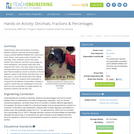Updating search results...

# 1 Result

View
Selected filters:Educational Use
Rating
0.0 stars

Students learn about and practice converting between fractions, decimals and percentages. Using a LEGO® MINDSTORMS® NXT robot and a touch sensor, each group inputs a fraction of its choosing. Team members convert this same fraction into a decimal, and then a percentage via hand calculations, and double check their work using the NXT robot. Then they observe the robot moving forward and record that distance. Students learn that the distance moved is a fraction of the full distance, based on the fraction that they input, so if they input ½, the robot moves half of the original distance. From this, students work backwards to compute the full distance. Groups then compete in a game in which they are challenged to move the robot as close as possible to a target distance by inputting a fraction into the NXT bot.

Subject:
Applied Science
Engineering
Mathematics
Technology
Material Type:
Activity/Lab
Provider:
TeachEngineering
Provider Set:
TeachEngineering
Author:
Javed Narain